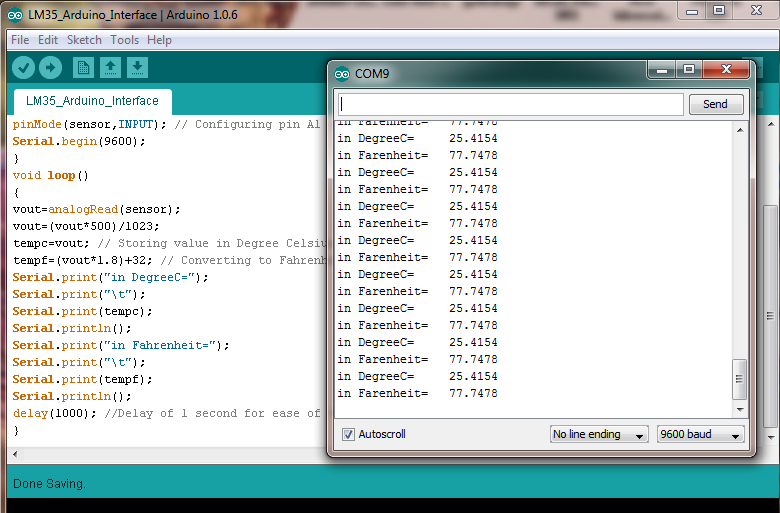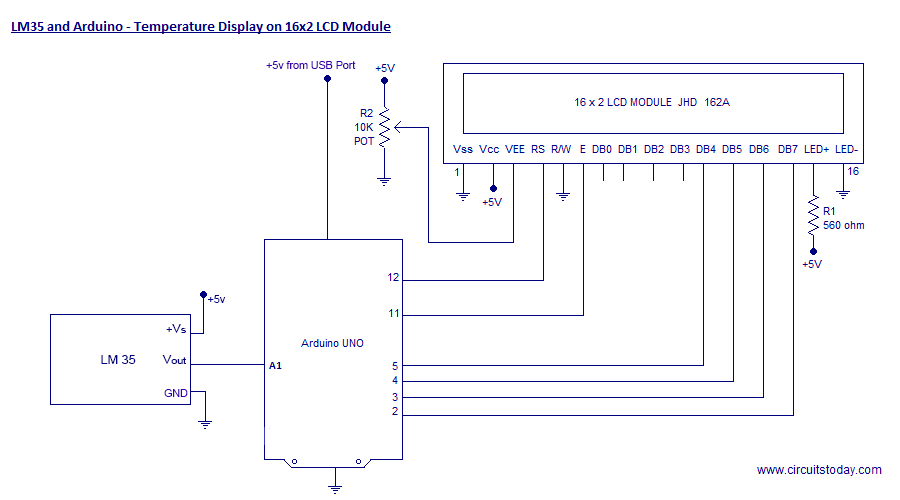# LM35 and Arduino – Temperature Measurement and Display on LCD

In this article, we are presenting a tutorial on how to interface LM35 and Arduino along with its program. Once we successfully interface arduino and lm35, we will go on to build a temperature display using arduino and a 16×2 LCD module which constantly monitors temperature around the measurement field/range of LM35 and displays the same on LCD module. So lets get to building the project!

LM35 is an analog, linear temperature sensor whose output voltage varies linearly with change in temperature. LM35 is three terminal linear temperature sensor from National semiconductors. It can measure temperature from-55 degree celsius to +150 degree celsius. The voltage output of the LM35 increases 10mV per degree Celsius rise in temperature. LM35 can be operated from a 5V supply and the stand by current is less than 60uA. The pin out of  LM35 is shown in the figure below.So that’s all info you need about LM35 for this particular temperature display project using arduino uno. So lets get to LM35 temperature sensor interfacing with arduino!

We are using Arduino Uno as our board and LM35 can be connected to arduino as shown in circuit diagram.

Note:- LM35 is an analog temperature sensor. This means the output of LM35 is an analog signal. Microcontrollers dont accept analog signals as their input directly. We need to convert this analog output signal to digital before we can feed it to a microcontroller’s input. For this purpose, we can use an ADC( Analog to Digital Converter).If we are using a basic microcontroller like 8051, we need to use an external ADC to convert analog output from LM35 to digital. We then feed the output of ADC ( converted digital value) to input of 8051. But modern day boards like Arduino and most modern day micro controllers come with inbuilt ADC. Our arduino uno has an in built 10 bit ADC (6 channel).If we are using a basic microcontroller like 8051, we need to use an external ADC to convert analog output from LM35 to digital. We then feed the output of ADC ( converted digital value) to input of 8051. But modern day boards like Arduino and most modern day micro controllers come with inbuilt ADC. Our arduino uno has an in built 10 bit ADC (6 channel).

#### This Post / Project can also be found using search terms:

• lm35 temperature sensor arduino pdf
• how to measure the temperature with arduino and display it on lcd
• temperature lm35 lcd arduino
• lm 35 lcd screen led speaker and motor